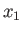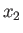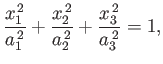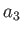Next: Analysis Up: Ellipsoidal Potential Theory Previous: Ellipsoidal Potential Theory

# Introduction

Let us adopt the right-handed Cartesian coordinate system,,. Consider a homogeneous ellipsoidal body whose outer boundary satisfies(D.1)

where,, andare the principal radii along the-,-, and-axes, respectively. Let us calculate the gravitational potential (i.e., the potential energy of a unit test mass) at some pointlying within this body. More information on ellipsoidal potential theory can be found in Chandrasekhar 1969.

Richard Fitzpatrick 2016-03-31